# Article overview: Deep Neural Networks Reveal a Gradient in the Complexity of Neural Representations across the Ventral Stream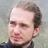PhD in Neuroscience and Artificial Intelligence. Machine Learning Architect at OffWorld.
Sep. 11, 2015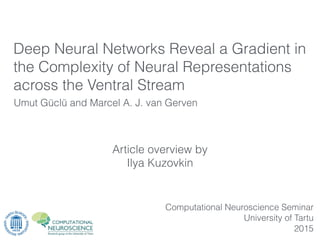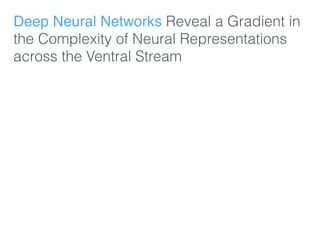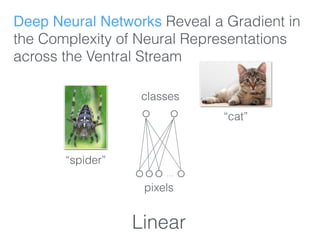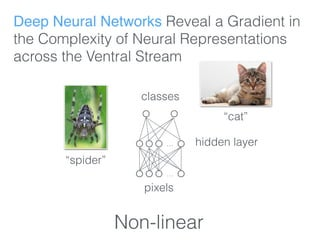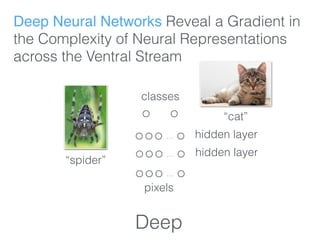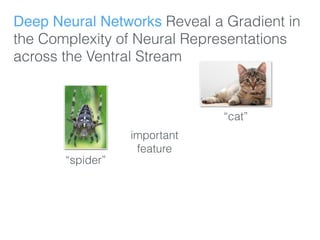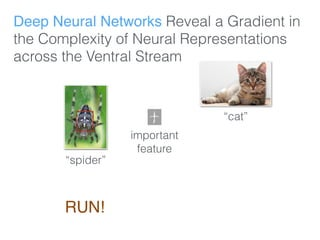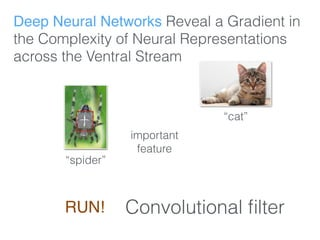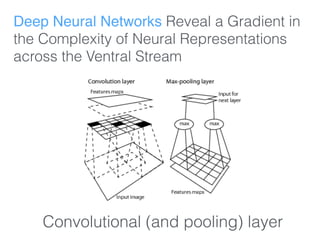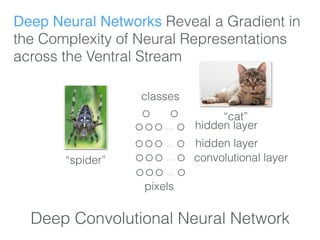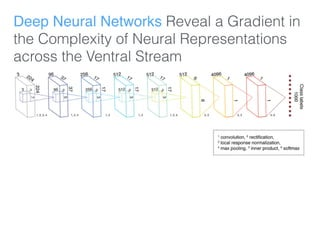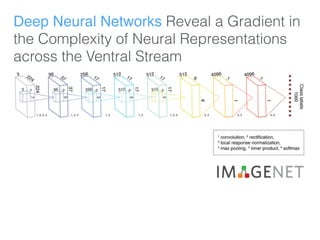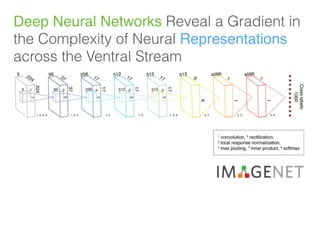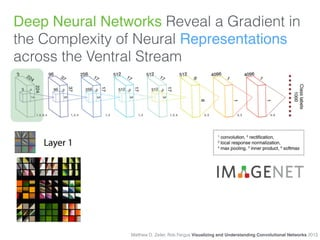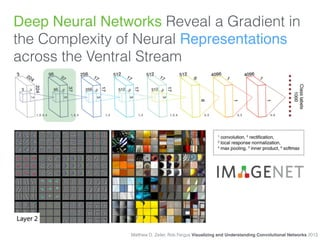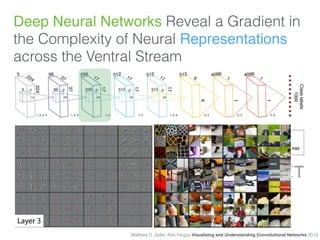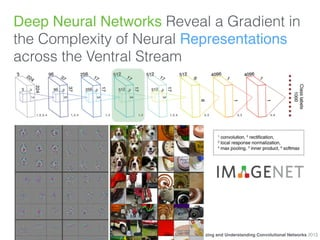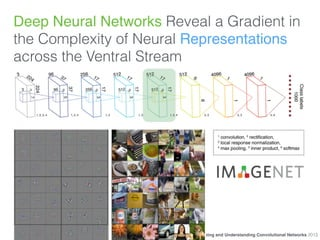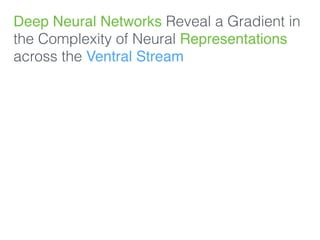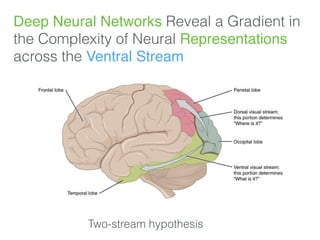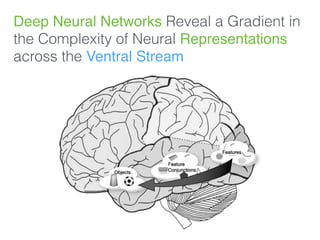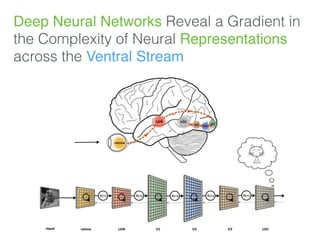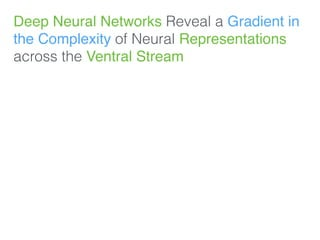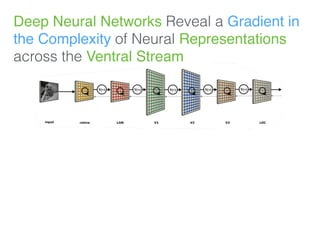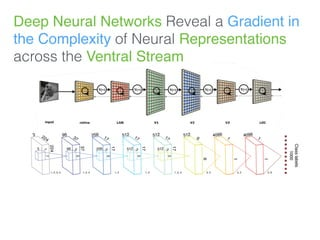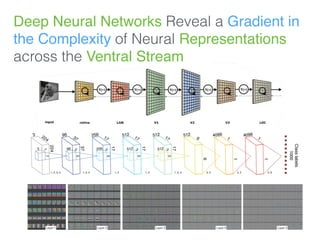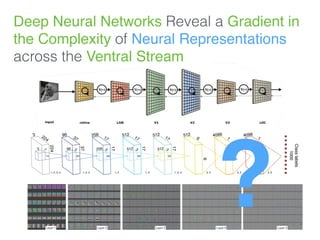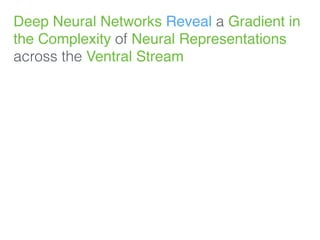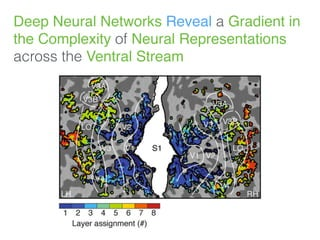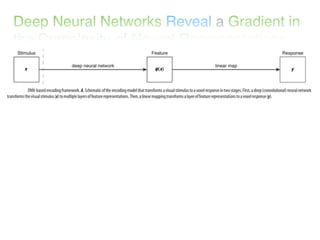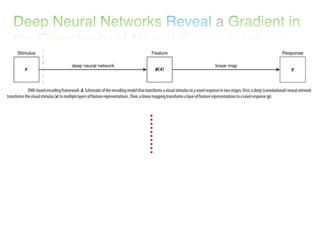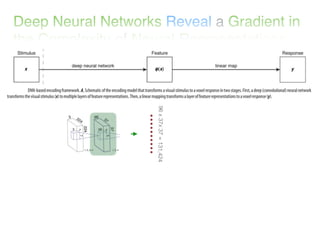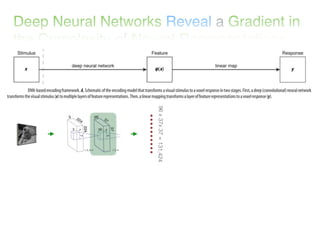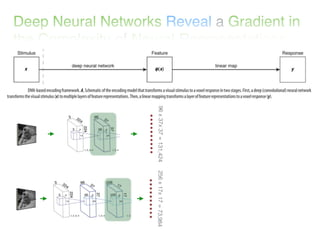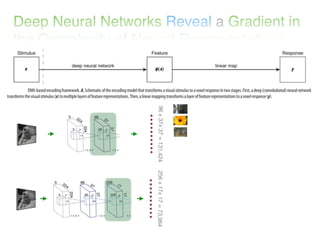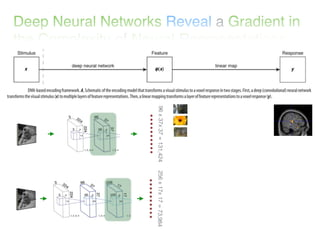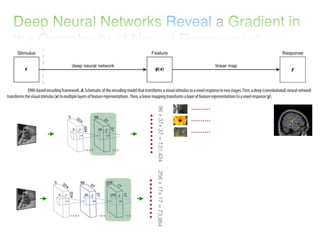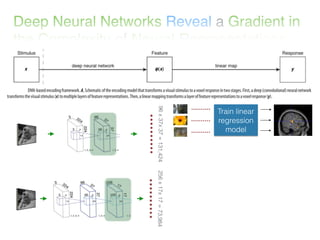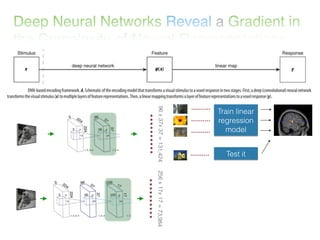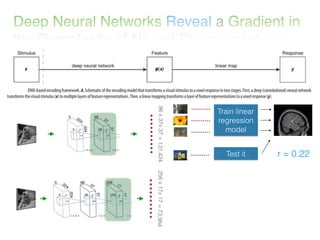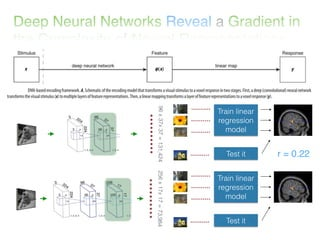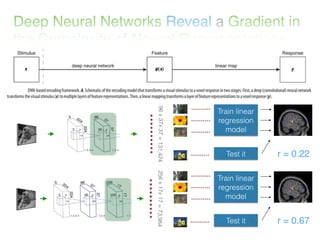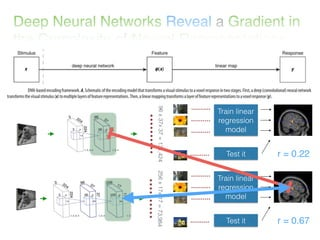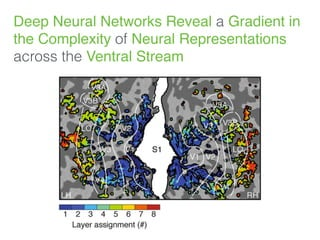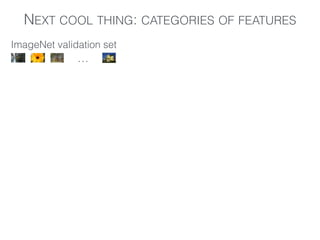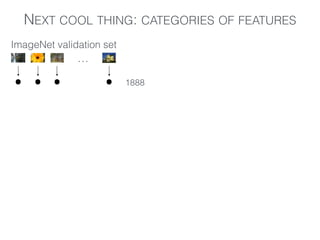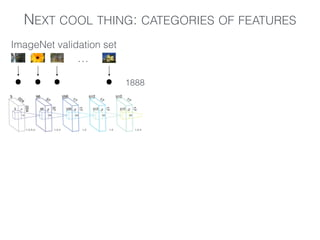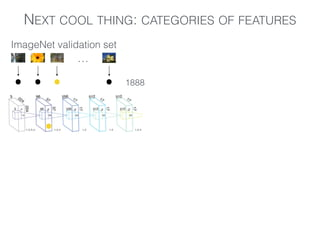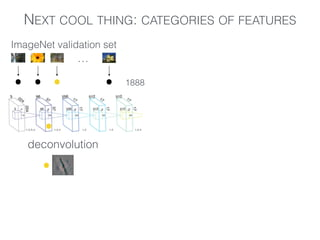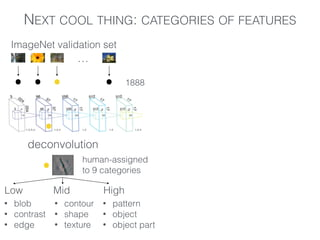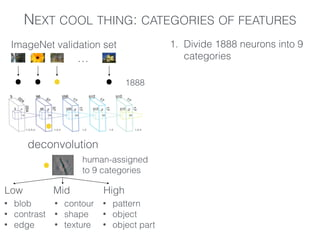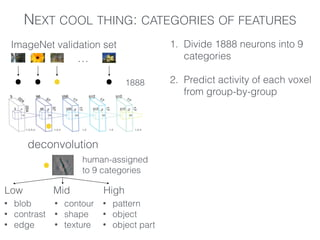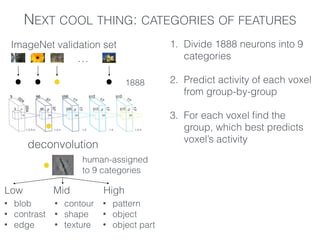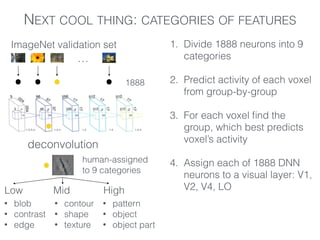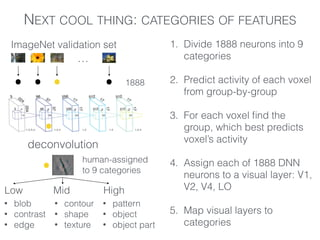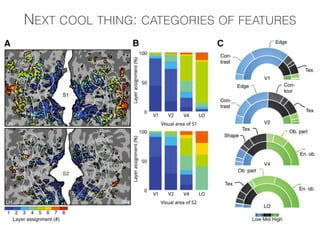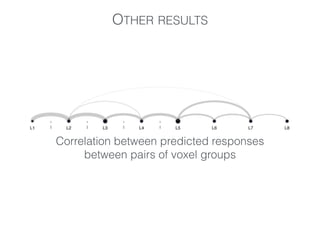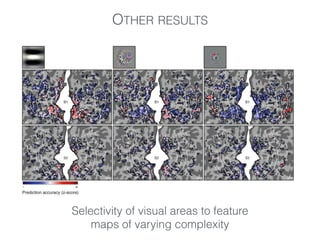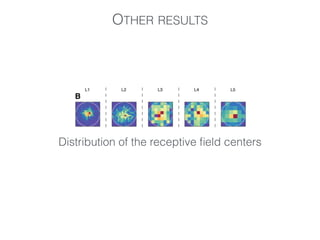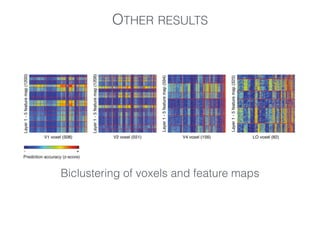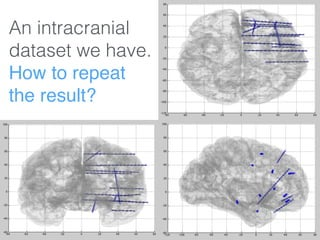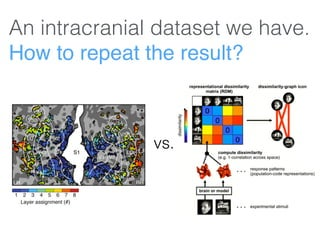1 of 63

### Article overview: Deep Neural Networks Reveal a Gradient in the Complexity of Neural Representations across the Ventral Stream

• 1. Article overview by Ilya Kuzovkin Umut Güclü and Marcel A. J. van Gerven Computational Neuroscience Seminar University of Tartu 2015 Deep Neural Networks Reveal a Gradient in the Complexity of Neural Representations across the Ventral Stream
• 2. Deep Neural Networks Reveal a Gradient in the Complexity of Neural Representations across the Ventral Stream
• 3. Deep Neural Networks Reveal a Gradient in the Complexity of Neural Representations across the Ventral Stream … pixels classes Linear “spider” “cat”
• 4. Deep Neural Networks Reveal a Gradient in the Complexity of Neural Representations across the Ventral Stream … pixels classes … hidden layer Non-linear “cat” “spider”
• 5. Deep Neural Networks Reveal a Gradient in the Complexity of Neural Representations across the Ventral Stream … pixels classes … hidden layer … hidden layer Deep “cat” “spider”
• 6. Deep Neural Networks Reveal a Gradient in the Complexity of Neural Representations across the Ventral Stream “spider” “cat” important feature
• 7. Deep Neural Networks Reveal a Gradient in the Complexity of Neural Representations across the Ventral Stream “spider” important feature RUN! “cat”
• 8. Deep Neural Networks Reveal a Gradient in the Complexity of Neural Representations across the Ventral Stream “spider” important feature RUN! Convolutional ﬁlter “cat”
• 9. Deep Neural Networks Reveal a Gradient in the Complexity of Neural Representations across the Ventral Stream Convolutional (and pooling) layer
• 10. Deep Neural Networks Reveal a Gradient in the Complexity of Neural Representations across the Ventral Stream … pixels classes … hidden layer … hidden layer … convolutional layer Deep Convolutional Neural Network “cat” “spider”
• 11. Deep Neural Networks Reveal a Gradient in the Complexity of Neural Representations across the Ventral Stream
• 12. Deep Neural Networks Reveal a Gradient in the Complexity of Neural Representations across the Ventral Stream
• 13. Deep Neural Networks Reveal a Gradient in the Complexity of Neural Representations across the Ventral Stream
• 14. Deep Neural Networks Reveal a Gradient in the Complexity of Neural Representations across the Ventral Stream Matthew D. Zeiler, Rob Fergus Visualizing and Understanding Convolutional Networks 2013
• 15. Matthew D. Zeiler, Rob Fergus Visualizing and Understanding Convolutional Networks 2013 Deep Neural Networks Reveal a Gradient in the Complexity of Neural Representations across the Ventral Stream
• 16. Matthew D. Zeiler, Rob Fergus Visualizing and Understanding Convolutional Networks 2013 Deep Neural Networks Reveal a Gradient in the Complexity of Neural Representations across the Ventral Stream
• 17. Matthew D. Zeiler, Rob Fergus Visualizing and Understanding Convolutional Networks 2013 Deep Neural Networks Reveal a Gradient in the Complexity of Neural Representations across the Ventral Stream
• 18. Matthew D. Zeiler, Rob Fergus Visualizing and Understanding Convolutional Networks 2013 Deep Neural Networks Reveal a Gradient in the Complexity of Neural Representations across the Ventral Stream
• 19. Deep Neural Networks Reveal a Gradient in the Complexity of Neural Representations across the Ventral Stream
• 20. Deep Neural Networks Reveal a Gradient in the Complexity of Neural Representations across the Ventral Stream Two-stream hypothesis
• 21. Deep Neural Networks Reveal a Gradient in the Complexity of Neural Representations across the Ventral Stream
• 22. Deep Neural Networks Reveal a Gradient in the Complexity of Neural Representations across the Ventral Stream
• 23. Deep Neural Networks Reveal a Gradient in the Complexity of Neural Representations across the Ventral Stream
• 24. Deep Neural Networks Reveal a Gradient in the Complexity of Neural Representations across the Ventral Stream
• 25. Deep Neural Networks Reveal a Gradient in the Complexity of Neural Representations across the Ventral Stream
• 26. Deep Neural Networks Reveal a Gradient in the Complexity of Neural Representations across the Ventral Stream
• 27. Deep Neural Networks Reveal a Gradient in the Complexity of Neural Representations across the Ventral Stream ?
• 28. Deep Neural Networks Reveal a Gradient in the Complexity of Neural Representations across the Ventral Stream
• 29. Deep Neural Networks Reveal a Gradient in the Complexity of Neural Representations across the Ventral Stream
• 32. 96x37x37=131,424
• 33. 96x37x37=131,424
• 34. 96x37x37=131,424256x17x17=73,984
• 35. 96x37x37=131,424256x17x17=73,984
• 36. 96x37x37=131,424256x17x17=73,984
• 37. 96x37x37=131,424256x17x17=73,984
• 38. 96x37x37=131,424256x17x17=73,984 Train linear regression model
• 39. 96x37x37=131,424256x17x17=73,984 Train linear regression model Test it
• 40. 96x37x37=131,424256x17x17=73,984 Train linear regression model Test it r = 0.22
• 41. 96x37x37=131,424256x17x17=73,984 Train linear regression model Test it r = 0.22 Train linear regression model Test it
• 42. 96x37x37=131,424256x17x17=73,984 Train linear regression model Test it r = 0.22 Train linear regression model Test it r = 0.67
• 43. 96x37x37=131,424256x17x17=73,984 Train linear regression model Test it r = 0.22 Train linear regression model Test it r = 0.67
• 44. Deep Neural Networks Reveal a Gradient in the Complexity of Neural Representations across the Ventral Stream
• 45. NEXT COOL THING: CATEGORIES OF FEATURES … ImageNet validation set
• 46. NEXT COOL THING: CATEGORIES OF FEATURES … ImageNet validation set ... . 1888
• 47. NEXT COOL THING: CATEGORIES OF FEATURES … ImageNet validation set ... . 1888
• 48. NEXT COOL THING: CATEGORIES OF FEATURES … ImageNet validation set ... . . 1888
• 49. NEXT COOL THING: CATEGORIES OF FEATURES … ImageNet validation set ... . . 1888 deconvolution .
• 50. NEXT COOL THING: CATEGORIES OF FEATURES … ImageNet validation set ... . . 1888 deconvolution . Low Mid High • blob • contrast • edge • contour • shape • texture • pattern • object • object part human-assigned to 9 categories
• 51. NEXT COOL THING: CATEGORIES OF FEATURES … ImageNet validation set ... . . 1888 deconvolution . Low Mid High • blob • contrast • edge • contour • shape • texture • pattern • object • object part human-assigned to 9 categories 1. Divide 1888 neurons into 9 categories
• 52. NEXT COOL THING: CATEGORIES OF FEATURES … ImageNet validation set ... . . 1888 deconvolution . Low Mid High • blob • contrast • edge • contour • shape • texture • pattern • object • object part human-assigned to 9 categories 1. Divide 1888 neurons into 9 categories ! 2. Predict activity of each voxel from group-by-group
• 53. NEXT COOL THING: CATEGORIES OF FEATURES … ImageNet validation set ... . . 1888 deconvolution . Low Mid High • blob • contrast • edge • contour • shape • texture • pattern • object • object part human-assigned to 9 categories 1. Divide 1888 neurons into 9 categories ! 2. Predict activity of each voxel from group-by-group ! 3. For each voxel ﬁnd the group, which best predicts voxel’s activity
• 54. NEXT COOL THING: CATEGORIES OF FEATURES … ImageNet validation set ... . . 1888 deconvolution . Low Mid High • blob • contrast • edge • contour • shape • texture • pattern • object • object part human-assigned to 9 categories 1. Divide 1888 neurons into 9 categories ! 2. Predict activity of each voxel from group-by-group ! 3. For each voxel ﬁnd the group, which best predicts voxel’s activity ! 4. Assign each of 1888 DNN neurons to a visual layer: V1, V2, V4, LO
• 55. NEXT COOL THING: CATEGORIES OF FEATURES … ImageNet validation set ... . . 1888 deconvolution . Low Mid High • blob • contrast • edge • contour • shape • texture • pattern • object • object part human-assigned to 9 categories 1. Divide 1888 neurons into 9 categories ! 2. Predict activity of each voxel from group-by-group ! 3. For each voxel ﬁnd the group, which best predicts voxel’s activity ! 4. Assign each of 1888 DNN neurons to a visual layer: V1, V2, V4, LO ! 5. Map visual layers to categories
• 56. NEXT COOL THING: CATEGORIES OF FEATURES
• 57. OTHER RESULTS Correlation between predicted responses between pairs of voxel groups
• 58. OTHER RESULTS Selectivity of visual areas to feature maps of varying complexity
• 59. OTHER RESULTS Distribution of the receptive ﬁeld centers
• 60. OTHER RESULTS Biclustering of voxels and feature maps
• 61. SUMMARY
• 62. An intracranial dataset we have. How to repeat the result?
• 63. An intracranial dataset we have. How to repeat the result? vs.